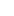# Tutor Hunt Questions

In a triangle ABC, side AB has length 10cm , side AC has length 5cm and angle BAC = theta( measured in degrees). The area of triangle is 15cm^2

A. Find the two possible values of cos theta?

Given that BC is the longest side of the triangle,
B.find the exact length of BC.
5 years ago

Please enter your response to the question below. The student will get a notification as soon your response has been approved by our moderation team.

To find cosθ;;, use the formula for the area of a triangle i.e. AREA=1/2 x a x b x sinC.=> For this case: 15= 1/2 x 10 x 5 x sinC to find sinC.=> SinC = 3/5 thus, Arcsin(3/5)=+- 4/5 or +-0.8
To find the exact length of BC, use the cosine rule.=> c(sq)=a(sq)+b(sq)-2abCosC=> c(sq)=10(sq)+5(sq)-2(10)(5)(+-4/5)=> c(sq)= Square root of 205

A

Using the formula for area of the triangle we get 0.5*10*5*sin(theta)=15 so sin(theta)=3/5. Then using the identity (sinx)^2+(cos^x)^2=1, we get (3/5)^2+(cos(theta))^2=1 so (cos(theta))^2=16/25 so cos(theta)=+- 4/5

B

Using the cosine rule we get BC^2 =5^2+10^2-2*5*10*cos(theta). If we draw the two possible triangles that could be formed, we see that one gives theta being obtuse and one gives theta as being acute. We are told BC is the longest side so theta must be obtuse and the value of cos(theta) that gives this is when cos(theta)=-4/5. So BC^2=125-100(-4/5)=125+80=205. So BC is of length sqrt(205) because it is a length we took the positive root.

Area of triangle = 1/2 x b x c x sin (BAC) [angle BAC = theta]
so 15 = 1/2 x 5 x 10 x sin (BAC) = 25 sin (BAC)
=> sin (BAC) = 15/25 = 3/5

trig identity: sin^2(x) + cos^2(x) = 1
=> cos^2(x) = 1 - sin^2(x)

Applying this:
cos^2(BAC) = 1 - (3/5)^2 = 16/25
=> cos(BAC) = +/- 4/5
so cos(theta) = +4/5 or -4/5 [+0.8 or -0.8]

Answered by Nigel | 11 months ago

A. Using the formula Area = 1/2(abSinC), we can rearrange this to get SinC = 2Area/ab
Plugging in the values for Area, a and b, we get SinTheta = 3/5
Using either a right angled triangle or cos^2x + sin^2x = 1, we end up with CosTheta = 4/5 or -4/5 (if you use the right angled triangle, symmetry property of CosX gets you -4/5)

B. Using the Cosine Rule: BC^2 = AB^2 + AC^2 - 2(AB)(AC)CosTheta
Using both values for CosTheta:
CosTheta = 4/5 gets you BC = 3√;;5

CosTheta = -4/5 gets you BC = √;;205

As BC is the longest side of the triangle, BC is √;;205cm as 3√;;5 is smaller than 10.